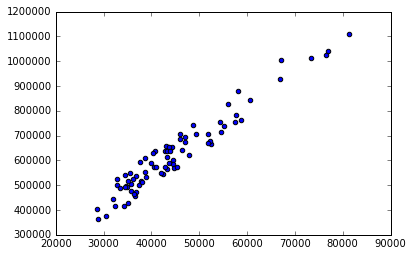• No products in the cart.

# 204.1.1 Correlation in Python

## Correlation

### What is need of correlation?

• Is there any association between hours of study and grades?
• Is there any association between number of temples in a city & murder rate?
• What happens to sweater sales with increase in temperature? What is the strength of association between them?
• What happens to ice-cream sales v.s temperature? What is the strength of association between them?
• How to quantify the association?
• Which of the above examples has very strong association?
• Correlation

## Correlation coefficient

• It is a measure of linear association.
• r is the ratio of variance together vs product of individual variances.
Correlationcoefficient(r)=CovarianceofXYSqrt(VarianceXVarianceY)
• Correlation 0 No linear association
• Correlation 0 to 0.25 Negligible positive association
• Correlation 0.25-0.5 Weak positive association
• Correlation 0.5-0.75 Moderate positive association
• Correlation >0.75 Very Strong positive association

### Practice : Correlation Calculation

• Dataset: AirPassengers\AirPassengers.csv
• Find the correlation between number of passengers and promotional budget.
• Draw a scatter plot between number of passengers and promotional budget
• Find the correlation between number of passengers and Service_Quality_Score
In :
import pandas as pd
air.shape

Out:
(80, 9)
In :
air.columns.values

Out:
array(['Week_num', 'Passengers', 'Promotion_Budget',
'Service_Quality_Score', 'Holiday_week',
'Delayed_Cancelled_flight_ind', 'Inter_metro_flight_ratio',
'Bad_Weather_Ind', 'Technical_issues_ind'], dtype=object)
In :
#Find the correlation between number of passengers and promotional budget.
import numpy as np
np.corrcoef(air.Passengers,air.Promotion_Budget)

Out:
array([[ 1.        ,  0.96585103],
[ 0.96585103,  1.        ]])
In :
#Draw a scatter plot between number of passengers and promotional budget
import matplotlib.pyplot as plt
%matplotlib inline
plt.scatter(air.Passengers, air.Promotion_Budget)

Out:
<matplotlib.collections.PathCollection at 0x8feb8d0>In :
#Find the correlation between number of passengers and Service_Quality_Score
np.corrcoef(air.Passengers,air.Service_Quality_Score)

Out:
array([[ 1.        , -0.88653002],
[-0.88653002,  1.        ]])

### Beyond Pearson Correlation

• Correlation coefficient measures for different types of data
 Variable Y\X Quantitative /Continuous X Ordinal/Ranked/Discrete X Nominal/Categorical X Quantitative Y Pearson r Biserial rb Point Biserial rpb Ordinal/Ranked/Discrete Y Biserial rb Spearman rho/Kendall’s Rank Biserial rrb Nominal/Categorical Y Point Biserial rpb Rank Biserial rrb Phi, Contingency Coeff, V

The next post is about regression in python.

Link to the next post : https://statinfer.com/204-1-2-regression-in-python/

21st June 2017